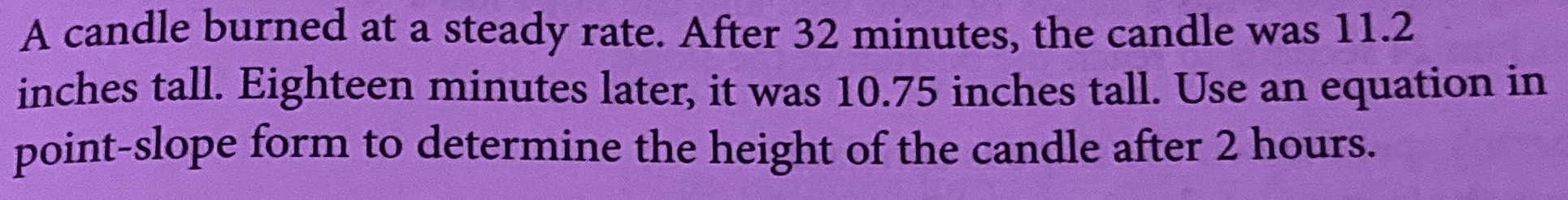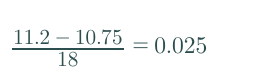### ¿Todavía tienes preguntas de matemáticas?

Pregunte a nuestros tutores expertos
Algebra
PreguntaA candle burned at a steady rate. After $$32$$ minutes, the candle was $$11.2$$ inches tall. Eighteen minutes later, it was $$10.75$$ inches tall. Use an equation in point-slope form to determine the height of the candle after $$2$$ hours.